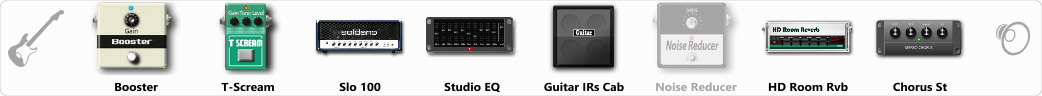# Sownd god

Discussion in 'ToneLib-GFX presets' started by Jean C.Guion, Jul 13, 2020.

1. Sownd god

Preset name: LYNCH [live drive]

i use a irig hd2 with the gain a just peaking orange not red , and the gain on the input side of the sim at about -21db..just lights up the first couple bars on the gain meter...I do this so as to have a realistic dynamic at the front end of the sim....
so you will have to set amp gain and or booster/screamer gain to your liking....

Effects chain:Effect: "Booster" (Dynamics / Filter), active - "yes"
"Gain" = 60

Effect: "T-Scream" (Overdrive / Distortion), active - "yes"
"Drive" = 27
"Tone" = 76
"Level" = 79

Effect: "Slo 100" (Amp simulators), active - "yes"
"Gain" = 71
"Bass" = 82
"Middle" = 76
"Treble" = 33
"Presence" = 40
"Master" = 70
"Output" = 50
"Level (dB)" = -3

Effect: "Studio EQ" (Dynamics / Filter), active - "yes"
"31 Hz" = -6
"62 Hz" = -2
"125 Hz" = 1
"250 Hz" = -2
"500 Hz" = 0
"1 kHz" = -2
"2 kHz" = 8
"4 kHz" = -13
"8 kHz" = -10
"16 kHz" = -14
"above 16 kHz" = 0
"Level (dB)" = 0

Effect: "Guitar IRs Cab" (Cabinets), active - "yes"
"Model" = Soldano Slant (4x12")
"Mic Position" = Middle
"Mic Distance" = Middle
"Low Cut (Hz)" = 39
"Hi Cut (kHz)" = 3.5
"Mix" = 100
"Level (dB)" = 5

Effect: "Noise Reducer" (Dynamics / Filter), active - "no"
"Sens" = 18
"Mode" = Soft

Effect: "HD Room Rvb" (Reverb), active - "yes"
"Time" = 7.8
"PreLPF" = 79
"PreDelay" = 135
"HiDamp" = 92
"LoGain" = -3.5
"Mix" = 40

Effect: "Chorus St" (Modulation / Sfx), active - "yes"
"Speed" = 1.9
"Depth" = 31
"Center" = 5.0
"Mix" = 39

Note: You will need to download and install the ToneLib-GFX software to use the preset.

File size:
695 bytes
Views:
2,959